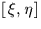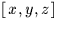Next: SLA_TP2V - Tangent Plane to Direction Cosines
Up: SUBPROGRAM SPECIFICATIONS
Previous: SLA_SVDSOL - Solution Vector from SVD

## SLA_TP2S - Tangent Plane to Spherical

ACTION:
Transform tangent plane coordinates into spherical coordinates (single precision)

CALL:
CALL sla_TP2S (XI, ETA, RAZ, DECZ, RA, DEC)

GIVEN:

 R XI,ETA tangent plane rectangular coordinates (radians) RAZ,DECZ R spherical coordinates of tangent point (radians)

RETURNED:

NOTES:
1.
The projection is called the gnomonic projection; the Cartesian coordinatesare called standard coordinates. The latter are in units of the distance from the tangent plane to the projection point, i.e. radians near the origin.
2.
When working inrather than spherical coordinates, the equivalent Cartesian routine sla_TP2V is available.Next: SLA_TP2V - Tangent Plane to Direction Cosines
Up: SUBPROGRAM SPECIFICATIONS
Previous: SLA_SVDSOL - Solution Vector from SVD

SLALIB --- Positional Astronomy Library